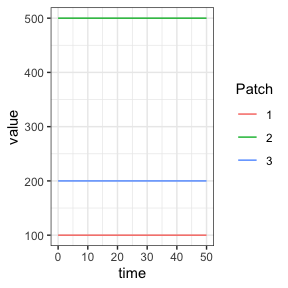# Basic Competition Aquatic Mosquito Model

The basic competition aquatic mosquito model fulfills the generic interface of the aquatic mosquito component. It has a single compartment “larvae” for each aquatic habitat, and mosquitoes in that aquatic habitat suffer density-independent and dependent mortality, and mature at some rate $$\psi$$.

# Differential Equations

Given $$\Lambda$$ and some egg laying rate from the adult mosquito population we could formulate and solve a dynamical model of aquatic mosquitoes to give that emergence rate. However, in the example here we will simply use a trace-based (forced) emergence model, so that $$\Lambda$$ completely specifies the aquatic mosquitoes.

The simplest model of aquatic (immature) mosquito dynamics with negative feedback (density dependence) is:

$\dot{L} = \eta - (\psi+\phi+\theta L)L$

Because the equations allow the number of larval habitats $$l$$ to differ from $$p$$, in general the emergence rate is given by:

$\Lambda = \mathcal{N}\cdot \alpha$

Where $$\mathcal{N}$$ is a $$p\times l$$ matrix and $$\alpha$$ is a length $$l$$ column vector given as:

$\alpha = \psi L$

# Equilibrium solutions

In general, if we know the value of $$\Lambda$$ at equilibrium we can solve for $$L$$ directly by using the above two equations. Then we can consider $$\theta$$, the strength of density dependence to be unknown and solve such that:

$\theta = (\eta - \psi L - \phi L) / L^2$ # Example

library(exDE)
library(deSolve)
library(data.table)
library(ggplot2)

Here we run a simple example with 3 aquatic habitats at equilibrium. We use exDE::make_parameters_L_basic to set up parameters. Please note that this only runs the aquatic mosquito component and that most users should read our fully worked example to run a full simulation.

nHabitats <- 3
alpha <- c(10, 50, 20)
eta <- c(250, 500, 170)
psi <- 1/10
phi <- 1/12

L <- alpha/psi
theta <- (eta - psi*L - phi*L)/(L^2)

params <- list(
nHabitats = nHabitats
)
params <- list2env(params)

make_parameters_L_basic(pars = params, psi = psi, phi = phi, theta = theta, L0 = L)
make_indices(params)

y0 <- L

out <- deSolve::ode(y = y0, times = 0:50, func = function(t, y, pars, eta) {
list(dLdt(t, y, pars, eta))
}, parms = params, method = 'lsoda', eta = eta)

colnames(out)[params$L_ix+1] <- paste0('L_', 1:params$nHabitats)

out <- as.data.table(out)
out <- melt(out, id.vars = 'time')
out[, c("Component", "Patch") := tstrsplit(variable, '_', fixed = TRUE)]
out[, variable := NULL]

ggplot(data = out, mapping = aes(x = time, y = value, color = Patch)) +
geom_line() +
theme_bw()